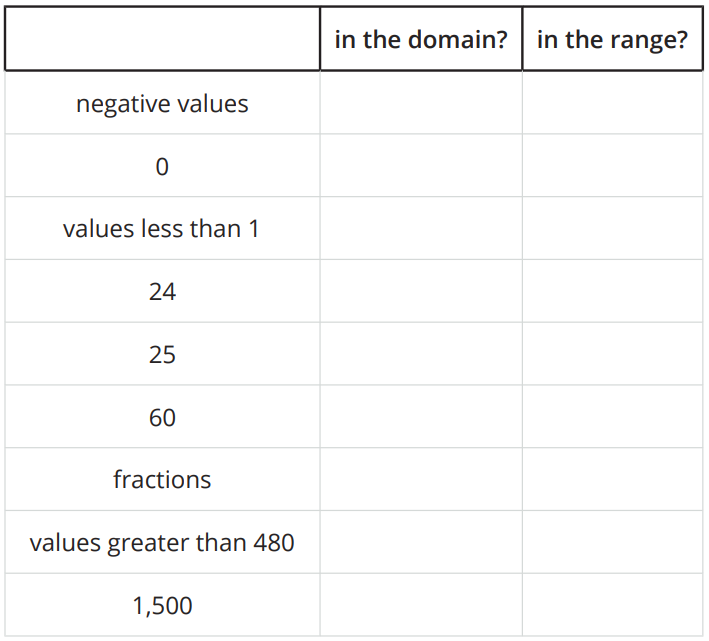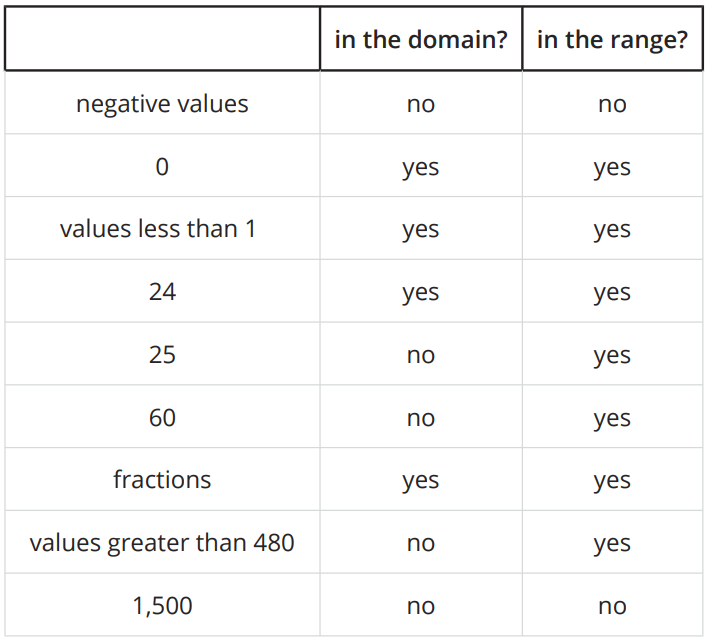# A.4.10.4 What Could Be the Trouble?

Consider the function f(x)=6/(x-2). To find out the sets of possible input and output values of the function, Clare created a table and evaluated f at some values of x. Along the way, she ran into some trouble. 1. Find f(x) for each x-value Clare listed.

Describe what Clare’s trouble might be.

2. Use graphing technology to graph function f. What do you notice about the graph?

3. Use a calculator to compute the value you and Clare had trouble computing. What do you notice about the computation?

4. How would you describe the domain of function f?

Tell students that function q gives the number of minutes a person sleeps as a function of the number of hours they sleep in a 24-hour period. Display a graphic organizer such as shown.Ask students to decide whether each value or set of values described in the first column could be in the domain and in the range of the function. They should be prepared to explain their decisions (some of which may depend on the assumptions they made about the situation). Once the class completes the organizer (an example is shown here), give students a moment to come up with a holistic description of the domain and range of this function.